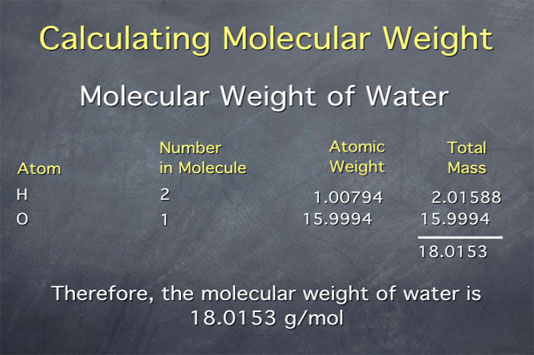# Stoichiometry Tutorials: Calculating Molecular Weight / Molar Mass

(from a complete OLI stoichiometry course)

The molecular weight is the mass of one mole of a substance. Usually, the units used for this are grams per mole. In this movie, we show how to calculate the molecular weight of a substance from the atomic weights given on the periodic table. On another page, we use the molecular weight to convert between the macroscopic scale (grams of a substance) and the microscopic scale (number of molecules of that substance).

### Calculating Molecular Weight Movie Text

We can calculate the molecular weight of a substance using its chemical formula and the periodic table.

Say that we want to calculate the molecular weight of water. The molecular weight is the sum of the atomic weights of the atoms in the molecule. We can go about our calculation by making a table. We list the atoms in the molecule down the left. Water contains hydrogen and oxygen. Then we write down how many of each of these atoms the molecule contains, which, in the case of water, is 2 and 1.We then take the atomic weight of the atoms from the periodic table. For hydrogen, the atomic weight is 1.00794, and for oxygen it is 15.9994. Since there are 2 hydrogens in the molecule, the total weight of hydrogen in water is 2 times 1.00794, or 2.01588. There is only 1 oxygen, so the total weight of oxygen is 15.9994.

Finally, we add up the weights of all the atoms to get the total molecular weight of water, 18.0153 grams per mole.

 Element Atomic Weight (g/mol) Ba 137.327 C 12.0107 Ca 40.078 Cr 51.9961 H 1.00794 Mg 24.3050 N 14.0067 Na 22.989770 O 15.9994 P 30.973761

Use the data from the periodic table shown above to answer the following questions.